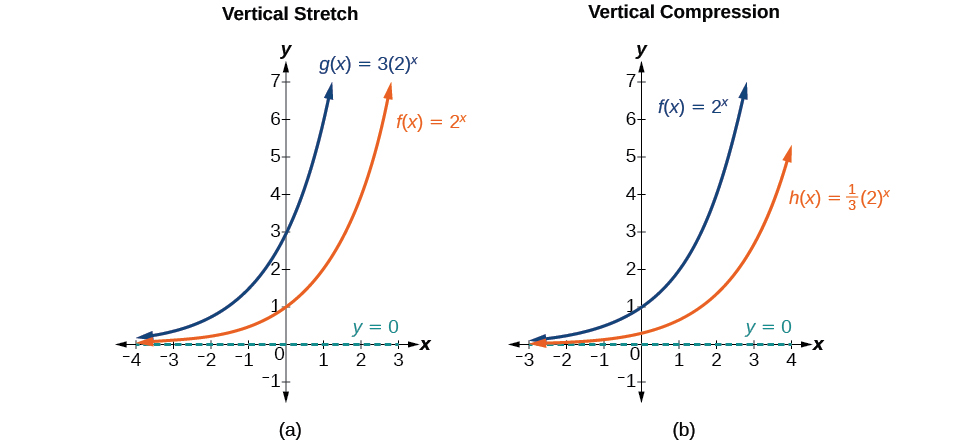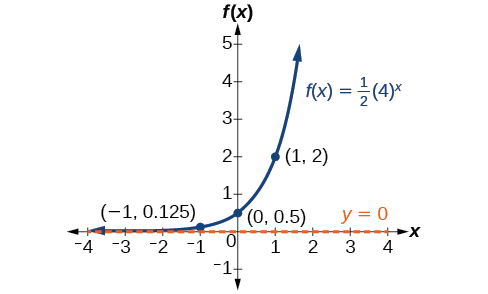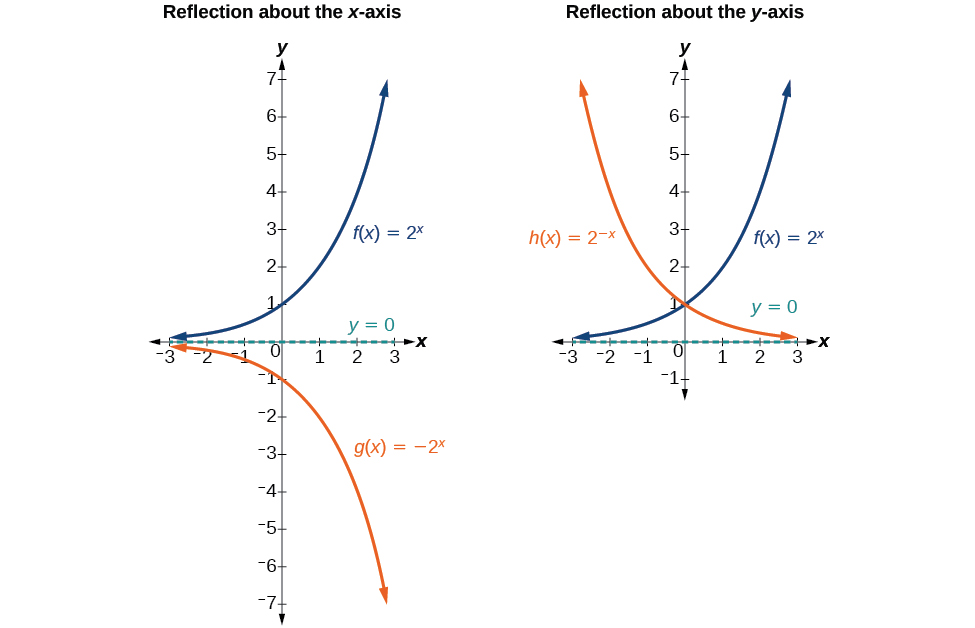# 6.2 Graphs of exponential functions  (Page 4/6)

 Page 4 / 6(a)   g ( x ) = 3 ( 2 ) x   stretches the graph of   f ( x ) = 2 x   vertically by a factor of   3.   (b)   h ( x ) = 1 3 ( 2 ) x   compresses the graph of   f ( x ) = 2 x   vertically by a factor of   1 3 .

## Stretches and compressions of the parent function f ( x ) = b x

For any factor $\text{\hspace{0.17em}}a>0,$ the function $\text{\hspace{0.17em}}f\left(x\right)=a{\left(b\right)}^{x}$

• is stretched vertically by a factor of $\text{\hspace{0.17em}}a\text{\hspace{0.17em}}$ if $\text{\hspace{0.17em}}|a|>1.$
• is compressed vertically by a factor of $\text{\hspace{0.17em}}a\text{\hspace{0.17em}}$ if $\text{\hspace{0.17em}}|a|<1.$
• has a y -intercept of $\text{\hspace{0.17em}}\left(0,a\right).$
• has a horizontal asymptote at $\text{\hspace{0.17em}}y=0,$ a range of $\text{\hspace{0.17em}}\left(0,\infty \right),$ and a domain of $\text{\hspace{0.17em}}\left(-\infty ,\infty \right),$ which are unchanged from the parent function.

## Graphing the stretch of an exponential function

Sketch a graph of $\text{\hspace{0.17em}}f\left(x\right)=4{\left(\frac{1}{2}\right)}^{x}.\text{\hspace{0.17em}}$ State the domain, range, and asymptote.

Before graphing, identify the behavior and key points on the graph.

• Since $\text{\hspace{0.17em}}b=\frac{1}{2}\text{\hspace{0.17em}}$ is between zero and one, the left tail of the graph will increase without bound as $\text{\hspace{0.17em}}x\text{\hspace{0.17em}}$ decreases, and the right tail will approach the x -axis as $\text{\hspace{0.17em}}x\text{\hspace{0.17em}}$ increases.
• Since $\text{\hspace{0.17em}}a=4,$ the graph of $\text{\hspace{0.17em}}f\left(x\right)={\left(\frac{1}{2}\right)}^{x}\text{\hspace{0.17em}}$ will be stretched by a factor of $\text{\hspace{0.17em}}4.$
• Create a table of points as shown in [link] .  $x$ $-3$ $-2$ $-1$ $0$ $1$ $2$ $3$ $f\left(x\right)=4\left(\frac{1}{2}{\right)}^{x}$ $32$ $16$ $8$ $4$ $2$ $1$ $0.5$
• Plot the y- intercept, $\text{\hspace{0.17em}}\left(0,4\right),$ along with two other points. We can use $\text{\hspace{0.17em}}\left(-1,8\right)\text{\hspace{0.17em}}$ and $\text{\hspace{0.17em}}\left(1,2\right).$

Draw a smooth curve connecting the points, as shown in [link] .

The domain is $\text{\hspace{0.17em}}\left(-\infty ,\infty \right);\text{\hspace{0.17em}}$ the range is $\text{\hspace{0.17em}}\left(0,\infty \right);\text{\hspace{0.17em}}$ the horizontal asymptote is $\text{\hspace{0.17em}}y=0.$

Sketch the graph of $\text{\hspace{0.17em}}f\left(x\right)=\frac{1}{2}{\left(4\right)}^{x}.\text{\hspace{0.17em}}$ State the domain, range, and asymptote.

The domain is $\text{\hspace{0.17em}}\left(-\infty ,\infty \right);\text{\hspace{0.17em}}$ the range is $\text{\hspace{0.17em}}\left(0,\infty \right);\text{\hspace{0.17em}}$ the horizontal asymptote is $\text{\hspace{0.17em}}y=0.\text{\hspace{0.17em}}$## Graphing reflections

In addition to shifting, compressing, and stretching a graph, we can also reflect it about the x -axis or the y -axis. When we multiply the parent function $\text{\hspace{0.17em}}f\left(x\right)={b}^{x}\text{\hspace{0.17em}}$ by $\text{\hspace{0.17em}}-1,$ we get a reflection about the x -axis. When we multiply the input by $\text{\hspace{0.17em}}-1,$ we get a reflection about the y -axis. For example, if we begin by graphing the parent function $\text{\hspace{0.17em}}f\left(x\right)={2}^{x},$ we can then graph the two reflections alongside it. The reflection about the x -axis, $\text{\hspace{0.17em}}g\left(x\right)={-2}^{x},$ is shown on the left side of [link] , and the reflection about the y -axis $\text{\hspace{0.17em}}h\left(x\right)={2}^{-x},$ is shown on the right side of [link] .(a)   g ( x ) = − 2 x   reflects the graph of   f ( x ) = 2 x   about the x-axis. (b)   g ( x ) = 2 − x   reflects the graph of   f ( x ) = 2 x   about the y -axis.

## Reflections of the parent function f ( x ) = b x

The function $\text{\hspace{0.17em}}f\left(x\right)=-{b}^{x}$

• reflects the parent function $\text{\hspace{0.17em}}f\left(x\right)={b}^{x}\text{\hspace{0.17em}}$ about the x -axis.
• has a y -intercept of $\text{\hspace{0.17em}}\left(0,-1\right).$
• has a range of $\text{\hspace{0.17em}}\left(-\infty ,0\right)$
• has a horizontal asymptote at $\text{\hspace{0.17em}}y=0\text{\hspace{0.17em}}$ and domain of $\text{\hspace{0.17em}}\left(-\infty ,\infty \right),$ which are unchanged from the parent function.

The function $\text{\hspace{0.17em}}f\left(x\right)={b}^{-x}$

• reflects the parent function $\text{\hspace{0.17em}}f\left(x\right)={b}^{x}\text{\hspace{0.17em}}$ about the y -axis.
• has a y -intercept of $\text{\hspace{0.17em}}\left(0,1\right),$ a horizontal asymptote at $\text{\hspace{0.17em}}y=0,$ a range of $\text{\hspace{0.17em}}\left(0,\infty \right),$ and a domain of $\text{\hspace{0.17em}}\left(-\infty ,\infty \right),$ which are unchanged from the parent function.

## Writing and graphing the reflection of an exponential function

Find and graph the equation for a function, $\text{\hspace{0.17em}}g\left(x\right),$ that reflects $\text{\hspace{0.17em}}f\left(x\right)={\left(\frac{1}{4}\right)}^{x}\text{\hspace{0.17em}}$ about the x -axis. State its domain, range, and asymptote.

Since we want to reflect the parent function $\text{\hspace{0.17em}}f\left(x\right)={\left(\frac{1}{4}\right)}^{x}\text{\hspace{0.17em}}$ about the x- axis, we multiply $\text{\hspace{0.17em}}f\left(x\right)\text{\hspace{0.17em}}$ by $\text{\hspace{0.17em}}-1\text{\hspace{0.17em}}$ to get, $\text{\hspace{0.17em}}g\left(x\right)=-{\left(\frac{1}{4}\right)}^{x}.\text{\hspace{0.17em}}$ Next we create a table of points as in [link] .

 $x$ $-3$ $-2$ $-1$ $0$ $1$ $2$ $3$ $g\left(x\right)=-\left(\frac{1}{4}{\right)}^{x}$ $-64$ $-16$ $-4$ $-1$ $-0.25$ $-0.0625$ $-0.0156$

Plot the y- intercept, $\text{\hspace{0.17em}}\left(0,-1\right),$ along with two other points. We can use $\text{\hspace{0.17em}}\left(-1,-4\right)\text{\hspace{0.17em}}$ and $\text{\hspace{0.17em}}\left(1,-0.25\right).$

Draw a smooth curve connecting the points:

The domain is $\text{\hspace{0.17em}}\left(-\infty ,\infty \right);\text{\hspace{0.17em}}$ the range is $\text{\hspace{0.17em}}\left(-\infty ,0\right);\text{\hspace{0.17em}}$ the horizontal asymptote is $\text{\hspace{0.17em}}y=0.$

how do I set up the problem?
what is a solution set?
Harshika
find the subring of gaussian integers?
Rofiqul
hello, I am happy to help!
Abdullahi
hi mam
Mark
find the value of 2x=32
divide by 2 on each side of the equal sign to solve for x
corri
X=16
Michael
Want to review on complex number 1.What are complex number 2.How to solve complex number problems.
Beyan
yes i wantt to review
Mark
use the y -intercept and slope to sketch the graph of the equation y=6x
how do we prove the quadratic formular
Darius
hello, if you have a question about Algebra 2. I may be able to help. I am an Algebra 2 Teacher
thank you help me with how to prove the quadratic equation
Seidu
may God blessed u for that. Please I want u to help me in sets.
Opoku
what is math number
4
Trista
x-2y+3z=-3 2x-y+z=7 -x+3y-z=6
can you teacch how to solve that🙏
Mark
Solve for the first variable in one of the equations, then substitute the result into the other equation. Point For: (6111,4111,−411)(6111,4111,-411) Equation Form: x=6111,y=4111,z=−411x=6111,y=4111,z=-411
Brenna
(61/11,41/11,−4/11)
Brenna
x=61/11 y=41/11 z=−4/11 x=61/11 y=41/11 z=-4/11
Brenna
Need help solving this problem (2/7)^-2
x+2y-z=7
Sidiki
what is the coefficient of -4×
-1
Shedrak
the operation * is x * y =x + y/ 1+(x × y) show if the operation is commutative if x × y is not equal to -1
An investment account was opened with an initial deposit of \$9,600 and earns 7.4% interest, compounded continuously. How much will the account be worth after 15 years?
lim x to infinity e^1-e^-1/log(1+x)
given eccentricity and a point find the equiationByByBy Janet ForresterBy Richley CrapoBy OpenStaxBy Michael SagBy Cath YuBy OpenStaxBy Jugnu KhanBy Cath YuBy OpenStaxBy Edgar Delgado